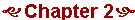# ALGEBRA

HomeThe four operations and their signs.
The function of parentheses.
Terms versus factors.
Powers and exponents.
The order of operations.
Values and evaluations.
Evaluating algebraic expressions.

The integers.
The algebraic sign and the absolute value.
Subtracting a larger number from a smaller.
The number line.
The negative of any number.

Naming terms. The rule for adding terms.
Subtracting a negative number.

The Rule of Signs.

The rule of symmetry. Commutative rules. Inverses.
Two rules for equations.

The definition of reciprocals. The definition of division. Rules for 0.

Parentheses. Brackets. Braces.
The relationship of  ba  to  ab.

The law of inverses.
Transposing.
A logical sequence of statements.
Simple fractional equations.

11.  Inequalities

Absolute value equations.
Absolute value inequalities.13.  Exponents I

Powers of a number.
Rules of exponents: When to add, when to multiply.

The definition of a polynomial in x.
Factoring polynomials.
Factoring by grouping.
Equations in which the unknown is a common factor.

Perfect square trinomials.
The square of a trinomial.
Completing the square.
Geometrical algebra.

Summary of Multiplying/Factoring.
Factoring by grouping.
The sum and difference of any two powers:  an ± bn.

20.  Exponents II

Negative exponents. Exponent 0. Scientific notation.Rational expressions. The principle of equivalent fractions. Reducing to lowest terms.

Complex fractions.

The Lowest Common Multiple (LCM) of a series of terms.

Clearing of fractions.

The whole is equal to the sum of the parts.
Same time problem: Upstream-downstream.
Total time problem. Job problem.Square roots.
Equations x² = a, and the principal square root.
Rationalizing a denominator.
Real numbers.Conjugate pairs.

Roots of numbers. The index of a radical.
Fractional exponents.
Negative exponents.The square root of a negative number.
The real and imaginary components.
Conjugate pairs.Straight lines.

The distance of a point from the origin.
The distance between any two points.
A proof of the Pythagorean theorem.

The equation of the first degree and its graph.
Vertical and horizontal lines.

The slope intercept form of the equation of a straight line. The general form.
Parallel and perpendicular lines.
The point-slope formula. The two-point formula.

The method of addition. The method of substitution. Cramer's Rule: The method of determinants.
Three equations in three unknowns.

Investment problems. Mixture problems.
Upstream-downstream problems.

Solution by factoring.
Completing the square.
The discriminant.
The graph of a quadratic: A parabola.38.  Logarithms

Definition. The three laws of logarithms.
Common logarithms.39.  Variation

Direct variation. The constant of proportionality.
Varies as the square. Varies inversely. Varies as the inverse square.